# Chapter10- Fluid Machinery¶

## Ex1-pg495¶

In :
import math
#calculate the impeller and torque and power
##Volume flow rate in gpm:
Q= 150.;
##Value of Vrb2 in ft/sec:
Vrb2=10.;
##Radius of outter impeller in inches:
R2=2.;
##Impeller Speed in rpm:
w=3450.;
##Density of air in slug/ft**3
p=1.94;
##input and power##

##Impeller exit width b2(in feet):
b2=Q*12./(2.*math.pi*R2*Vrb2*7.48*60.)
##Torque of the Shaft, Tshaft(in ft-lbf):
Tshaft=w*R2**2*p*Q*2.*math.pi/3600/7.48/144.
##Power, Wm(in hp):
Wm=w*Tshaft*2.*math.pi/60./550.
print ("RESULTS")
print'%s %.2f %s'% ("Impeller exit width: ",b2," feet")
print'%s %.2f %s'% ("Torque input: ",Tshaft," ft-lbf")
print'%s %.2f %s'% ("Power: ",Wm," hp")

RESULTS
Impeller exit width:  0.03  feet
Torque input:  6.51  ft-lbf
Power:  4.27  hp


## Ex2-pg497¶

In :
import math
#calculate volume flow rate  and torque and power
##Tip Diameter in metres:
Dt=1.1;
##Hub Diameter in metres:
Dh=0.8;
##Operating Speed in rpm:
w=1200.;
##Absolute inlet angle in degrees:
alpha1=30.;
betta1=30.;
betta2=60.;
##Density of air in kg/m**3
p=1.23;
##volume and power##

U=0.5*(Dh+Dt)/2.*1200.*2.*math.pi/60.
k=math.tan(alpha1/57.3)+(1./(math.cos(betta1/57.3))/(math.sin(betta1/57.3)))
Vn1=U/k
V1=Vn1/math.cos(alpha1/57.3)
Vt1=V1*math.sin(alpha1/57.3)
Vrb1=Vn1/math.sin(betta1/57.3)
##Volume flow rate (in m**3/sec):
Q=math.pi/4.*Vn1*(Dt**2.-Dh**2.)
k=(U-Vn1*(1./(math.cos(betta1/57.3))/(math.sin(betta1/57.3))))
k1=k/Vn1
alpha2= math.tan(k)*57.3
V2=Vn1/math.cos(alpha2/57.3)
Vt2=V2*math.sin(alpha2/57.3)
##Rotor Torque (in N-m):
Tz=p*Q*(Dh+Dt)/4.*(Vt2-Vt1)
##Power required (in W):
Wm=w*2*math.pi/60.*Tz
print ("RESULTS")
print'%s %.2f %s'% ("Volume flow rate: ",Q," m^3/sec")
print'%s %.2f %s'% ("Rotor Torque: ",Tz," N-m")
print'%s %.2f %s'% ("Power required: ",Wm," W")

RESULTS
Volume flow rate:  9.26  m^3/sec
Rotor Torque:  -164.33  N-m
Power required:  -20649.89  W


## Ex3-pg504¶

In :
import math
#calculate pump charstics
import numpy
%matplotlib inline
import warnings
warnings.filterwarnings('ignore')
#Rate of flow in gm:
Q=numpy.array([0, 500 ,800, 1000, 1100, 1200 ,1400, 1500]);
#Suction pressure in psig:
ps=numpy.array([ 0.65, 0.25 ,-0.35, -0.92, -1.24, -1.62, -2.42, -2.89]);
#Discharge pressure in psig:
pd=numpy.array([53.3, 48.3 ,42.3, 36.9, 33 ,27.8, 15.3, 7.3]);
#Motor Current in amps:
I=numpy.array([18, 26.2 ,31 ,33.9, 35.2, 36.3, 38 ,39]);
#Acceleration due to gravity in ft/s^2:
g=32.2;
#Value of Zs in feet
zs=1;
#Density of air in slug/ft^3:
px=1.94;
#Value of ZD in feet:
zd=3.;
#Density of fluid in slug/ft^3:
py=1000.;
#Motor Efficiency:
Effm=0.9;
#Motor Supply in volts:
E=460.;
#Power Factor:
PF=0.875;
#Pump Power
Q=numpy.linspace(0,1500,num=8);
mQ=len(Q);
ps=numpy.linspace(-2.89,0.65,num=8);
mps=len(ps);
p1=numpy.zeros(mps)
p2=numpy.zeros(mps)

Hp=numpy.zeros(mps)
Wh=numpy.zeros(mps)
pd=numpy.linspace(7.3,53.3,num=8);
mpd=len(pd);
p2=numpy.zeros(mpd)
I=numpy.linspace(18,39,num=8)
mI=len(I);
Pin=numpy.zeros(mI)
Effp=numpy.zeros(mI)
#correct measured static pressures to he pump centreline p1, p2(in psig):
for j in  range (1,mps):
p1[j]=ps[j]+px*g*zs/144.
p2=numpy.zeros(mps)
for j in  range (mpd):
p2[j]=pd[j]+px*g*zd/144.
#The value of Pump head(in feet):
p2=numpy.zeros(mps)
for j1 in range (1,mps):
Hp[j]=p2[j1]-p1[j]/(px*g)*144.
#Values of Hydraulic Power delivered(in hp):
for j in range (1,mps):
Wh[j]=Q[j]*(p2[j]-p1[j])/(7.48/60)*(144./550.)
#Values of motor power output(in hp):
for j in range(1,mI):
Pin[j]=Effm*math.sqrt(3)*PF*E*I[j]/746.
#Values of Pump Efficiecy:
for j in range (1,mI):
Effp[j]= Wh[j]/Pin[j]*100.
#Plotting pump characteristics:
pyplot.plot(Q,Hp)
pyplot.title('Pump Characteristics')
pyplot.show()
pyplot.plot(Q,Pin)
pyplot.title('Pump Characteristics')
pyplot.show
pyplot.plot(Q,Effp)

pyplot.title('Pump Characteristics')
pyplot.show()
#,'Volume flow rate(in gpm)',['Pump Efficincy(%)    ','   Pump Head(in feet)   ','    Pump Power input(in hp)   '])
#legend('Hp','Pin','Effp')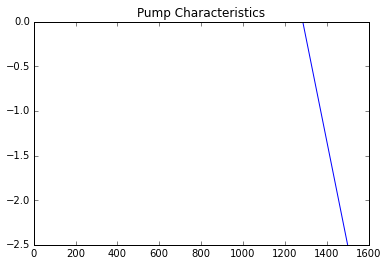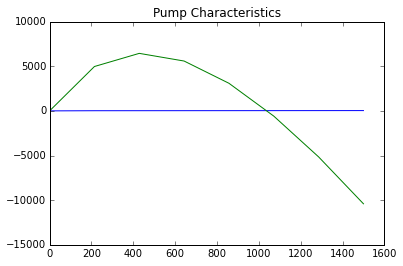## Ex6-pg518¶

In :
import math
#calculate specific speed
Hus=21.9;
##Volume flow rate in US customary units:
Qus=300.;
##Working seed in rpm:
N=1170.;
##Aceleration due to graviy in m/s**2
g=9.81;
##Specific and relation##

##Specific speed in Us customary units:
Nscu=N*Qus**0.5/Hus**0.75
##Conversion to SI units:
w=1170.*2.*math.pi/60.;
Qsi=Qus/7.48/60*0.305**3.;
Hsi=Hus*0.305;
##Energy per unit mass is:
h=g*Hsi;
##Specific speed in SI units:
Nssi=w*Qsi**0.5/h**0.75
##Conversion to hertz:
whz=N/60.;
##Specific speed in European units:
Nseu=whz*Qsi**0.5/65.5**0.75
##Relation between specific speeds in Us customary units and European units:
Conversionfactor1=Nscu/Nseu
##Relation between specific speeds in Us customary units and SI units:
Conversionfactor2=Nscu/Nssi
print("RESULTS")
print'%s %.2f %s'% ("Specific speed in US customary units: ",Nscu," ")
print'%s %.2f %s'% ("Specific speed in SI units: ",Nssi," ")
print'%s %.2f %s'% ("Specific speedin European units: ",Nseu," ")
print'%s %.2f %s'% ("Relation between specific speeds in Us customary units and European units: ",Conversionfactor1," ")
print'%s %.2f %s'% ("Relation between specific speeds in Us customary units and SI units: ",Conversionfactor2," ")

RESULTS
Specific speed in US customary units:  2001.77
Specific speed in SI units:  0.73
Specific speedin European units:  0.12
Relation between specific speeds in Us customary units and European units:  17162.31
Relation between specific speeds in Us customary units and SI units:  2732.28


## Ex7-pg522¶

In :
import numpy
import math
#calculate relation b/w pump and head
%matplotlib inline
import warnings
warnings.filterwarnings('ignore')
import math

from math import log
import numpy
##Volume flow rate(in gpm) at shut off condition for N1:
Q1so=0.;
##Volume flow(in gpm) rate at best efficiency for N1:
Q1be=300.;
##Head(in feet) at shut off condition for N1:
H1so=25.;
##Head(in feet) at best efficiency condition for N1:
H1be=21.9
##Operation Speed 1:
N1=1170.;
##Operation speed 2:
N2=1750.;

##Volume flow rate(in gpm) at shut off condition for N2:
Q2so=N2/N1*Q1so
##Volume flow(in gpm) rate at best efficiency for N2:
Q2be=N2/N1*Q1be
##Head(in feet) at shut off condition for N2:
H2so=(N2/N1)**2*H1so
##Head(in feet) at best efficiency condition for N2:
H2be=(N2/N1)**2*H1be
Q1=numpy.array([Q1so, Q1be]);
Q2=numpy.array([Q2so, Q2be]);
H1=numpy.array([H1so, H1be]);
H2=numpy.array([H2so, H2be]);
pyplot.plot(Q1,H1)
pyplot.plot(Q2,H2)
pyplot.title('Comparison of head for both conditions')

Out:
<matplotlib.text.Text at 0x5a80750>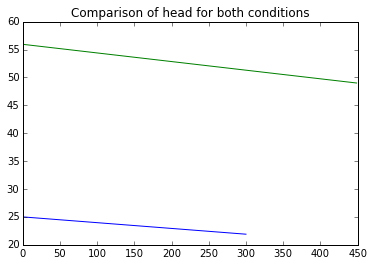## Ex8-pg525¶

In :
##For 5 inch nominal pipe line, diameter D:
import numpy
import math
import matplotlib
from matplotlib import pyplot
%matplotlib inline
import warnings
warnings.filterwarnings('ignore')
#calculate suction head vs flow rate  and plot it
Di=5.047;
##Length of pipeline(in feet):
L=6.;
##Operatng spped (in rpm):
N=1750.;
##Water level abovepump centreline(in feet):
h=3.5;
##Temperature 1 of water(in Farenheit):
T1=80.;
##Temperature 2 of water (in Farenheit):
T2=180.;
##Volume flow rate of water(in gpm):
Q=1000.;
##Minor loss Coefficients:
K=0.5; SE=30; OGV=8;
##Atmospheric pressure(in lbf/in**2):
patm=14.7;
##Density of air(slug/ft**3):
p=1.93;
##Acceleration due to gravity(in ft/sec**2):
g=32.2;
##Head(in feet) due to vapor pressure of water for T =80F:
Hv1=1.17;
##Head(in feet) due to vapor pressure of water for T =180F:
Hv2=17.3;
##Kinematic viscosity of water at 80F:
v=0.927e-5;
##Value of discharges for plotting NPSHR(in gpm):
Qh=numpy.array([500 ,700, 900, 1100, 1300])
##Values of NPSHR obtained from Fig. D3 of appendix D:
NPSHRp=numpy.array([7, 8, 9.5, 12, 16])
##NPSHA and NPSHR##

##Diameter of pipe (in feet):
Df= Di/12.
##Area of crossection of pipe(in ft**2):
A=math.pi/4.*Df**2.
##Velocity of flow(in ft/sec):
V=Q/7.48/A/60.
##For water at T=80F,viscosity=0.927e-5 ft**2/sec, Reynolds number:
Re=V*Df/v
##Friction loss Coefficient for this value of Re:
f=0.0237;
##For cast iron, roughness(in feet):
e=0.00085
##e/D is:
e/Df
HL=K+f*(SE+OGV)+f*(L/Df)+1.
H1=patm*144./(p*g)
Vh=V**2./2./g
Hs=H1+h-HL*Vh
##NPSHA(in feet):
NPSHA=Hs+Vh-Hv1
##For a flow rate of 1000 gpm,NPSHR(in feet) for water at 80 F
NPSHR=10.
##PLOTTING NPSHA AND NPSHR VERSUS VOLUME FLOW RATE:
##For 80 F
Qp=numpy.linspace(0,1500,num=16);
mQp=len(Qp);
Vp=numpy.zeros(mQp)
Vhp=numpy.zeros(mQp)
Hs=numpy.zeros(mQp)
NPSHAp1=numpy.zeros(mQp)
NPSHAp2=numpy.zeros(mQp)
for j in range (1,mQp):
Vp[j]=Qp[j]/(7.48*A*60.);
Vhp[j]=(Vp[j]**2./2.)/g;
Hs[j]=H1+h-HL*Vhp[j];

for j in range (1,mQp):
NPSHAp1[j]=Hs[j]+(Vhp[j])-Hv1;

pyplot.plot(Qp,NPSHAp1)

pyplot.show()
pyplot.plot(Qh,NPSHRp)
pyplot.title('Volume flow rate(gpm)')
print("\n\nType (Resume) to continue or (abort) to end\n\n")
pyplot.legend('NPSHA','NPSHR')
pyplot.show()

##For 180 F
for j in range (1,mQp):
NPSHAp2[j]=Hs[j]+(Vhp[j])-Hv2;
pyplot.plot(Qp,NPSHAp2)
pyplot.show()
pyplot.plot(Qh,NPSHRp)
pyplot.title('Volume flow rate(gpm)')
pyplot.legend('NPSHA','NPSHR')
pyplot.show()
print("\n\nRESULTS\n\n")
print("\n\nNPSHA at Q=1000 gpm of water at 80 F: %.2f ft\n\n",NPSHA)
print("\n\nNPSHR at Q=1000 gpm of water at 80 F: %.1f ft\n\n",NPSHR)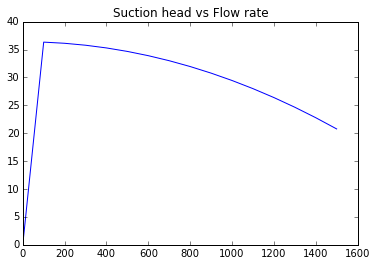Type (Resume) to continue or (abort) to end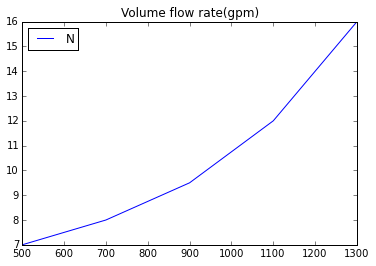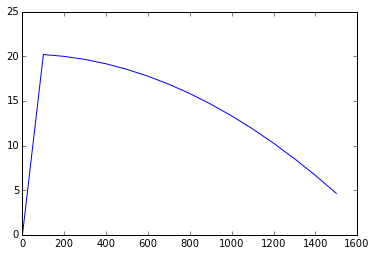RESULTS

('\n\nNPSHA at Q=1000 gpm of water at 80 F: %.2f ft\n\n', 29.447152524872912)
('\n\nNPSHR at Q=1000 gpm of water at 80 F: %.1f ft\n\n', 10.0)


## Ex11-pg546¶

In :
import numpy

import math
import matplotlib
from matplotlib import pyplot
%matplotlib inline
import warnings
warnings.filterwarnings('ignore')
#calculate performance curve for give variables and plot it
##Diameter of fan 1 (in inches):
D1=36;
##Operating speed of fan 1(in rpm):
N1=600
##Density of air used in fan 1(in lbm/ft**3):
d1=0.075;
##Diameter of fan 2(in inches):
D2=42;
##Operating speed of fan 2(in rpm):
N2=1150;
##Density of aif usd in fan 2(in lbm/ft**3):
d2= 0.045;
##The following values are obtained from the given graph
##Values of volume flow rate(in cfm) through fan 1:
Q1= numpy.array([0, 10000, 20000, 30000, 40000, 50000, 60000]);
##Values of pressure( in inches of H2O):
p1=numpy.array([ 3.68 ,3.75 ,3.50 ,2.96 ,2.12 ,1.02 ,0]);
##Values of power(in hp):
P1=numpy.array([ 11.1, 15.1 ,18.6 ,21.4 ,23.1 ,23.1, 21.0]);
##Efficiency (in %):
Eff=numpy.array([0, 37, 59, 65, 57 ,34 ,0]);
Q1=numpy.linspace(0,60000,num=7);
mQ= len(Q1);
Q2=numpy.zeros(mQ)
p1=numpy.linspace(0,3.7,num=7);
mp= len(p1);
p2=numpy.zeros(mp)
P1=numpy.linspace(11.1,23.1,num=7);
mP= len(P1);
P2=numpy.zeros(mP)
#Volume flow rate for fan 2(in cfm):
for j in range (1, mQ):
Q2[j]=Q1[j]*(N2/N1)*(D2/D1)**3
#Pressure values for fan 2(in inches of H2O):
for j in range (1,mp):
p2[j]=p1[j]*(d2/d1)*((N2/N1)**2.)*((D2/D1)**2.)
#Power values for fan 2(in hp):
for j in range (1,mP):
P2[j]=P1[j]*(d2/d1)*((N2/N1)**3.)*((D2/D1)**5.)
pyplot.plot(Q2,p2)
pyplot.title('Performance curves')
#,'Volume flow rate(in cfm)','Pressure head(in inches of water)')
print("\n\nType (resume) to continue or (abort) to exit\n\n")
pyplot.show()
pyplot.plot(Q2,P2)
pyplot.title('Performance curves')
#,'Volume flow rate(in cfm)','Power(in hp)')
print("\n\nType (resume) to continue or (abort) to exit\n\n")
pyplot.show()
pyplot.plot(Q2,Eff)
pyplot.title('Performance curves')
#,'Volume flow rate(in cfm)','Eficiency(in percentage)')
#Specific speed of fan(in US customary units) at operating point:
Nscu= 1150*110000**0.50*0.045**0.75/7.4**0.75
#Specific speed of fan (in SI units) at operating point:
Nssi=120*3110**0.5*0.721**0.75/1.84e3**0.75


Type (resume) to continue or (abort) to exit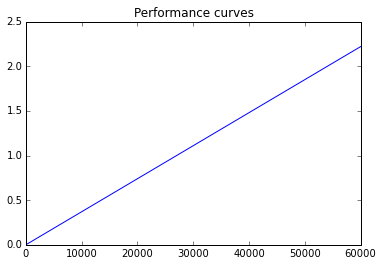Type (resume) to continue or (abort) to exit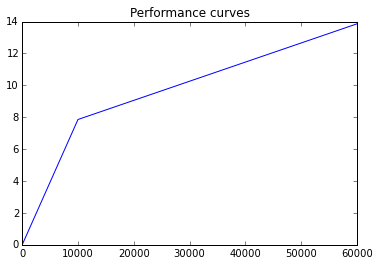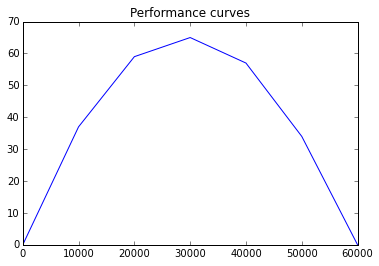## Ex12-pg552¶

In :
##Operation speed(in rpm):
#calculate volumeflow rate and torque and power required
N=2000.;
##Volume flow rate(in gpm):
Q=20.;
##Pressure(in psig):
p=1500.;
##Actual Pump Displacement(in##3/rev):
va=5.9;
##Volume flow rate at operating condition(in gpm):
Qo=46.5;
##Volume flow rate at maximum delivery(in gpm):
Qe=48.5;
##Pressure at operation condition(in psi):
po1=1500.;
##Efficiency of pump at operating condition:
Effp=0.84;
##Pressure at operating condition case 2(in psig):
po2=3000.;
##Power required##

##From given graph, for maximum delivery condition, Q=48.5gpm.
##Volume of oil per revolution delivered by the pump(in in##3/rev):
vc=Qe/N*231.
##Volumetric Effciency of pump at max flow:
Effv=vc/va
##Operating point of the pump is found to be at 1500 psig,Q=46.5gpm
##Power delivered by the fluid(in hp):
Pf=Qo*po1/(7.48/60.)*(44./550.)
##Input power(in hp):
Pi=Pf/Effp
##The power delivered to the load(in hp):
Pl=Q*(po1)/(7.48/60.)*(144./550.)
##Power dissipated by throttling(in hp):
Pd=Pf-Pl
##The dissipation with the variable displacement pump(in hp):
Pvd=Q*(po2-po1)/(7.48/60.)*(144./550.)
##Power required for te load sensing pump if pump pressure is 100psi above that required by the load(in hp):
Pls=Q*100/(7.48/60)*(144./550.)
print("RESULTS")
print'%s %.2f %s'% ("Volume of oil per revolution delivered by the pump: ",vc," in**3/rev")
print'%s %.2f %s'% ("Required pump power input: ",Pi," hp")
print'%s %.2f %s'% ("Power deliverd to the load: ",Pl," hp")
print'%s %.2f %s'% ("Power dissipated by throttling: ",Pd," hp")
print'%s %.2f %s'% ("The dissipation with the variable displacement pump: ",Pvd," hp")
print'%s %.2f %s'% ("Power required for te load sensing pump if pump pressure is 100psi above that required by the load: ",Pls," hp")

RESULTS
Volume of oil per revolution delivered by the pump:  5.60  in**3/rev
Required pump power input:  53284.95  hp
Power deliverd to the load:  63004.38  hp
Power dissipated by throttling:  -18245.02  hp
The dissipation with the variable displacement pump:  63004.38  hp
Power required for te load sensing pump if pump pressure is 100psi above that required by the load:  4200.29  hp


## Ex14-pg558¶

In :
import math
#calculate the operating speed of propeller and single speed power propeller
##Total propulsion power requirement(in MW):
P=11.4;
##From the given curves,Value of coefficients atoptimum efficiency are as follows:
J=0.85;
##Thrust Coefficient:
Cf=0.1;
##Torque Coefficint:
Ct=0.02;
##Efficiency:
Eff=0.66;
##Velocity of ship(in m/sec):
V=6.69;
##Density of water(in kg/m**3):
p=1025.;
##propeller##
##Propeller Thrust(in MN) :
Ft=P/V
##Required power input  to the propeller(in MW):
Pin=P/Eff
##Calculating value of D(in m):
nD=V/J
D=(Ft*10**6./p/(nD)**2./Cf)**0.5
##Operating speed (in rpm) is given by:
n=nD/D*60.
print ("RESULTS")
print'%s %.2f %s'% ("Diameter of the single propeller required to pwer the ship:",D," m")
print'%s %.2f %s'% ("Operating speed of the propeller: ",n," rpm")

RESULTS
Diameter of the single propeller required to pwer the ship: 16.38  m
Operating speed of the propeller:  28.83  rpm


## Ex16-pg569¶

In :
import math
#calculate the Tip speed ratio of windmill and Actual Thrust
##Diameter of windmill(in m):
D=26.;
##Operating speed(in rpm):
N=20.;
##Wind speed(in km/hr):
V=36.;
##Power Output(in W):
Po=41000.;
##Maximum efficiency occurs in following conditions:
##Efficiency:
Eff=0.593;
##Inteference Factor:
a=1./3.;
##Density of air(in kg/m**3):
p=1.23;
##Actual##

##Tip speed ratio of windmill:
X=N*2.*math.pi/60.*D/2./(V*5./18.)
##Accounting for whirl,max attainable efficiency is:
Efw=0.53;
##Kinetic energy flux(in W) is given by:
KEF=0.5*p*(V*5./18.)**3.*math.pi*(D/2.)**2.
##Actual Efficiency:
Effa=Po/KEF
##The maximum possible thrust occurs for an interference factor of:
amax=0.5;
##Thrust(in W):
Kx=p*(V*5./18.)**2.*math.pi*(D/2.)**2.*2.*amax*(1-amax)
print("RESULTS")
print'%s %.2f %s'% ("Tip speed ratio of windmill:",X,"")
print'%s %.2f %s'% ("Actual Efficiency: ",Effa,"")
print'%s %.2f %s'% ("Actual Thrust: ",Kx," W")

RESULTS
Tip speed ratio of windmill: 2.72
Actual Efficiency:  0.13
Actual Thrust:  32652.14  W